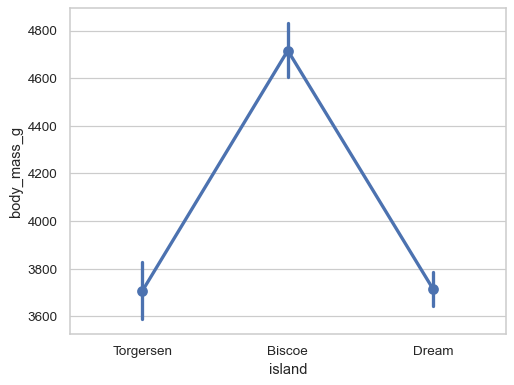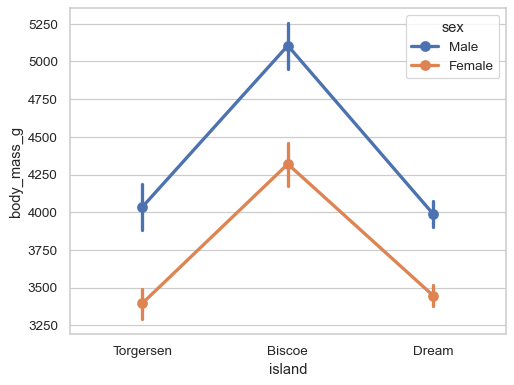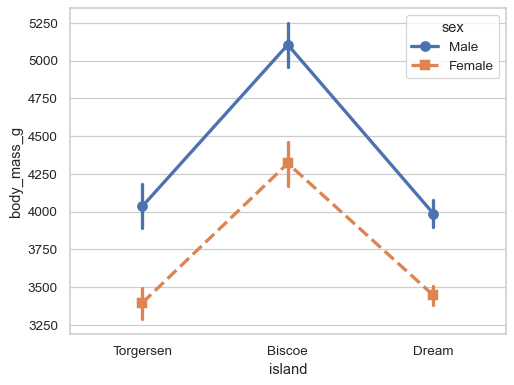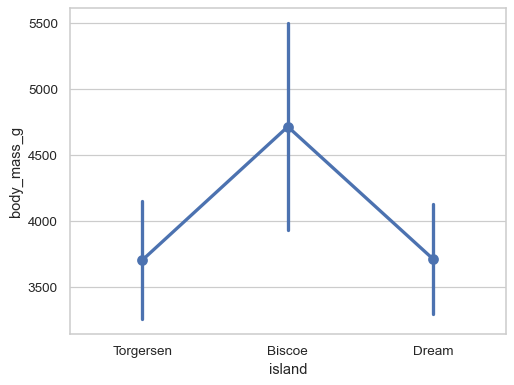# seaborn.pointplot#

seaborn.pointplot(data=None, *, x=None, y=None, hue=None, order=None, hue_order=None, estimator='mean', errorbar=('ci', 95), n_boot=1000, units=None, seed=None, markers='o', linestyles='-', dodge=False, join=True, scale=1, orient=None, color=None, palette=None, errwidth=None, ci='deprecated', capsize=None, label=None, ax=None)#

Show point estimates and errors using dot marks.

A point plot represents an estimate of central tendency for a numeric variable by the position of the dot and provides some indication of the uncertainty around that estimate using error bars.

Point plots can be more useful than bar plots for focusing comparisons between different levels of one or more categorical variables. They are particularly adept at showing interactions: how the relationship between levels of one categorical variable changes across levels of a second categorical variable. The lines that join each point from the same `hue` level allow interactions to be judged by differences in slope, which is easier for the eyes than comparing the heights of several groups of points or bars.

It is important to keep in mind that a point plot shows only the mean (or other estimator) value, but in many cases it may be more informative to show the distribution of values at each level of the categorical variables. In that case, other approaches such as a box or violin plot may be more appropriate.

Note

This function always treats one of the variables as categorical and draws data at ordinal positions (0, 1, … n) on the relevant axis, even when the data has a numeric or date type.

Parameters:
dataDataFrame, array, or list of arrays, optional

Dataset for plotting. If `x` and `y` are absent, this is interpreted as wide-form. Otherwise it is expected to be long-form.

x, y, huenames of variables in `data` or vector data, optional

Inputs for plotting long-form data. See examples for interpretation.

order, hue_orderlists of strings, optional

Order to plot the categorical levels in; otherwise the levels are inferred from the data objects.

estimatorstring or callable that maps vector -> scalar, optional

Statistical function to estimate within each categorical bin.

errorbarstring, (string, number) tuple, callable or None

Name of errorbar method (either “ci”, “pi”, “se”, or “sd”), or a tuple with a method name and a level parameter, or a function that maps from a vector to a (min, max) interval, or None to hide errorbar.

n_bootint, optional

Number of bootstrap samples used to compute confidence intervals.

unitsname of variable in `data` or vector data, optional

Identifier of sampling units, which will be used to perform a multilevel bootstrap and account for repeated measures design.

seedint, numpy.random.Generator, or numpy.random.RandomState, optional

Seed or random number generator for reproducible bootstrapping.

markersstring or list of strings, optional

Markers to use for each of the `hue` levels.

linestylesstring or list of strings, optional

Line styles to use for each of the `hue` levels.

dodgebool or float, optional

Amount to separate the points for each level of the `hue` variable along the categorical axis.

joinbool, optional

If `True`, lines will be drawn between point estimates at the same `hue` level.

scalefloat, optional

Scale factor for the plot elements.

orient“v” | “h”, optional

Orientation of the plot (vertical or horizontal). This is usually inferred based on the type of the input variables, but it can be used to resolve ambiguity when both `x` and `y` are numeric or when plotting wide-form data.

colormatplotlib color, optional

Single color for the elements in the plot.

palettepalette name, list, or dict

Colors to use for the different levels of the `hue` variable. Should be something that can be interpreted by `color_palette()`, or a dictionary mapping hue levels to matplotlib colors.

errwidthfloat, optional

Thickness of error bar lines (and caps).

capsizefloat, optional

Width of the “caps” on error bars.

labelstring, optional

Label to represent the plot in a legend, only relevant when not using `hue`.

axmatplotlib Axes, optional

Axes object to draw the plot onto, otherwise uses the current Axes.

Returns:
axmatplotlib Axes

Returns the Axes object with the plot drawn onto it.

`barplot`

Show point estimates and confidence intervals using bars.

`catplot`

Combine a categorical plot with a `FacetGrid`.

Examples

Group by a categorical varaible and plot aggregated values, with confidence intervals:

```df = sns.load_dataset("penguins")
sns.pointplot(data=df, x="island", y="body_mass_g")
```Add a second layer of grouping:

```sns.pointplot(data=df, x="island", y="body_mass_g", hue="sex")
```Adjust the artists along the categorical axis to reduce overplotting:

```sns.pointplot(data=df, x="sex", y="bill_depth_mm", hue="island", dodge=True)
```Use the error bars to show the standard deviation rather than a confidence interval:

```sns.pointplot(data=df, x="island", y="body_mass_g", errorbar="sd")
```Customize the appearance of the plot:

```sns.pointplot(
data=df, x="body_mass_g", y="island",
errorbar=("pi", 100), capsize=.4, join=False, color=".5",
)
```